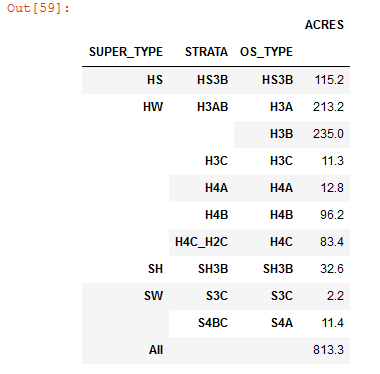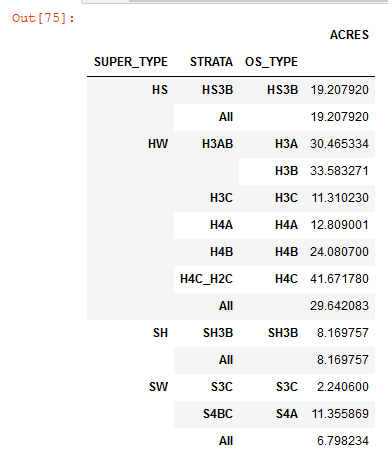# Pandas Pivot Table with rounding

15042
4
11-09-2018 08:21 AMOccasional Contributor III

I'm attempting to add in subtotals to a pivot table from a very basic array. The first example I provide I derived on my own, but this has no subtotals for each group.

The second example I borrowed and honestly I don't really get how it works just yet, and I cannot get a round to work.

``pd.pivot_table(df.round({'ACRES':1}),values = 'ACRES', index = ['SUPER_TYPE','STRATA', 'OS_TYPE'], aggfunc=np.sum, margins = True)‍‍``

Returns:2nd example:

``df.groupby(['SUPER_TYPE']).apply(lambda sub_df: sub_df.pivot_table(index=['STRATA', 'OS_TYPE'], values=['ACRES'], margins=True) )‍‍``Tags (1)
1 Solution

Accepted SolutionsbyMVP Legendary Contributor

then there are examples on Stack Exchange

python - Rounding entries in a Pandas DafaFrame - Stack Overflow  This one has a pivot table as an example

Numpy to the rescue!!

4 RepliesbyMVP Legendary Contributor

then there are examples on Stack Exchange

python - Rounding entries in a Pandas DafaFrame - Stack Overflow  This one has a pivot table as an example

Numpy to the rescue!!Occasional Contributor III

Dan Patterson, thanks for that...I didn't know it would be so simple. Here is what I came up with for my test code before I roll it up into a function. I've always got open ears for improvement! Edit: my pivot table fails to include a grand total. Any suggestions?

``````table = "\\\\fileServer\\MAP_PROJECTS\\LV_WEB\\SDE_CONNECTIONS\\LV_NEXUS.sde\\LV_NEXUS.DATAOWNER.NORTHEAST\\LV_NEXUS.DATAOWNER.NE_HARVEST_OPS"
HUID = "669-NMTC-139"
whereClause = """ "LV_HARVEST_UNIT_ID" = '{0}' """.format(HUID)
print(whereClause)
tableArray = arcpy.da.TableToNumPyArray(table, ['STAND_NUMB', 'SUPER_TYPE','STRATA', 'OS_TYPE', 'SILV_PRES', 'ACRES'], where_clause = whereClause)
df = pd.DataFrame(tableArray)
report = df.groupby(['SUPER_TYPE']).apply(lambda sub_df: sub_df.pivot_table(index=['STRATA', 'OS_TYPE', 'STAND_NUMB', 'SILV_PRES'], values=['ACRES'], margins=True) )
np.round(report,1)‍‍‍‍‍‍‍‍‍‍‍‍‍‍‍‍``````byMVP Legendary Contributor

Zachary

I have a few toolset post on the Code sharing site, I don't use pandas, preferring numpy instead, but feel free to examine coding.

Table Tools, has a crosstab/pivot table equivalent, amongst other thingsOccasional Contributor III

Dan Patterson‌ thanks! there's some real gold in there. I've bookmarked these and downloaded your table tools.

I ended up getting some help on SE and found an easy way to move the totals. Thanks again Dan!